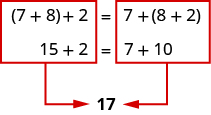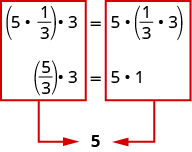## Using the Commutative and Associative Properties

### Learning Outcomes

• Identify the associative and commutative properties of addition and multiplication
• Use the associative and commutative properties of addition and multiplication to rewrite algebraic expressions

For some activities we perform, the order of certain processes does not matter, but the order of others do. For example, it does not make a difference if we put on the right shoe before the left or vice-versa. However, it does matter whether we put on shoes or socks first. The same thing is true for addition and multiplication.

## The Commutative Properties

The commutative property of addition states that numbers may be added in any order without affecting the sum.

$a+b=b+a$

We can better see this relationship when using real numbers.

Think about adding two numbers, such as $5$ and $3$.

$\begin{array}{cccc}\hfill 5+3\hfill & & & \hfill 3+5\hfill \\ \hfill 8\hfill & & & \hfill 8\hfill \end{array}$

The results are the same. $5+3=3+5$

### Example

Show that numbers may be added in any order without affecting the sum. $\left(-2\right)+7=5$

Notice, the order in which we add does not matter. The same is true when multiplying $5$ and $3$.

$\begin{array}{cccc}\hfill 5\cdot 3\hfill & & & \hfill 3\cdot 5\hfill \\ \hfill 15\hfill & & & \hfill 15\hfill \end{array}$

Again, the results are the same! $5\cdot 3=3\cdot 5$. The order in which we multiply does not matter.
Similarly, the commutative property of multiplication states that numbers may be multiplied in any order without affecting the product.

$a\cdot b=b\cdot a$

Again, consider an example with real numbers.

### Example

Show that numbers may be multiplied in any order without affecting the product.$\left(-11\right)\cdot\left(-4\right)=44$

These examples illustrate the commutative properties of addition and multiplication.

### Commutative Properties

Commutative Property of Addition: if $a$ and $b$ are real numbers, then

$a+b=b+a$

Commutative Property of Multiplication: if $a$ and $b$ are real numbers, then

$a\cdot b=b\cdot a$

The commutative properties have to do with order. If you change the order of the numbers when adding or multiplying, the result is the same.

### example

Use the commutative properties to rewrite the following expressions:
1. $-1+3=$
2. $4\cdot 9=$

Solution:

 1. $-1+3=$ Use the commutative property of addition to change the order. $-1+3=3+\left(-1\right)$
 2. $4\cdot 9=$ Use the commutative property of multiplication to change the order. $4\cdot 9=9\cdot 4$

### try it

What about subtraction? Does order matter when we subtract numbers? Does $7 - 3$ give the same result as $3 - 7?$

$\begin{array}{ccc}\hfill 7 - 3\hfill & & \hfill 3 - 7\hfill \\ \hfill 4\hfill & & \hfill -4\hfill \\ & \hfill 4\ne -4\hfill & \end{array}$
The results are not the same. $7 - 3\ne 3 - 7$

Since changing the order of the subtraction did not give the same result, we can say that subtraction is not commutative.

Let’s see what happens when we divide two numbers. Is division commutative?

$\begin{array}{ccc}\hfill 12\div 4\hfill & & \hfill 4\div 12\hfill \\ \hfill \frac{12}{4}\hfill & & \hfill \frac{4}{12}\hfill \\ \hfill 3\hfill & & \hfill \frac{1}{3}\hfill \\ & \hfill 3\ne \frac{1}{3}\hfill & \end{array}$

The results are not the same. So $12\div 4\ne 4\div 12$

Since changing the order of the division did not give the same result, division is not commutative.

Addition and multiplication are commutative. Subtraction and division are not commutative.## The Associative Properties

Suppose you were asked to simplify this expression.

$7+8+2$

Some people would think $7+8\text{ is }15$ and then $15+2\text{ is }17$. Others might start with $8+2\text{ makes }10$ and then $7+10\text{ makes }17$.

Both ways give the same result, as shown below. (Remember that parentheses are grouping symbols that indicate which operations should be done first.)When adding three numbers, changing the grouping of the numbers does not change the result. This is known as the Associative Property of Addition.

The associative property of addition tells us that numbers may be grouped differently without affecting the sum.
$a+\left(b+c\right)=\left(a+b\right)+c$

This property can be especially helpful when dealing with negative integers. Consider this example.

### Example

Show that regrouping addition does not affect the sum. $[15+\left(-9\right)]+23=29$

The same principle holds true for multiplication as well. Suppose we want to find the value of the following expression:

$5\cdot \frac{1}{3}\cdot 3$

Changing the grouping of the numbers gives the same result.When multiplying three numbers, changing the grouping of the numbers does not change the result. This is known as the Associative Property of Multiplication.

If we multiply three numbers, changing the grouping does not affect the product.

The associative property of multiplication tells us that it does not matter how we group numbers when multiplying. We can move the grouping symbols to make the calculation easier, and the product remains the same.

$a\left(bc\right)=\left(ab\right)c$

Consider this example.

### Example

Show that you can regroup numbers that are multiplied together and not affect the product.$\left(3\cdot4\right)\cdot5=60$

You probably know this, but the terminology may be new to you. These examples illustrate the Associative Properties.

### Associative Properties

Associative Property of Addition: if $a,b$, and $c$ are real numbers, then

$\left(a+b\right)+c=a+\left(b+c\right)$

Associative Property of Multiplication: if $a,b$, and $c$ are real numbers, then

$\left(a\cdot b\right)\cdot c=a\cdot \left(b\cdot c\right)$

### example

Use the associative properties to rewrite the following:

1. $\left(3+0.6\right)+0.4=$
2. $\left(-4\cdot \frac{2}{5}\right)\cdot 15=$

### try it

Are subtraction and division associative? Review these examples.

### Example

Use the associative property to explore whether subtraction and division are associative.

1) $8-\left(3-15\right)\stackrel{?}{=}\left(8-3\right)-15$

2) $64\div\left(8\div4\right)\stackrel{?}{=}\left(64\div8\right)\div4$

## Contribute!

Did you have an idea for improving this content? We’d love your input.